2019年09月12日

## 前言## bias 偏差 和 variance 方差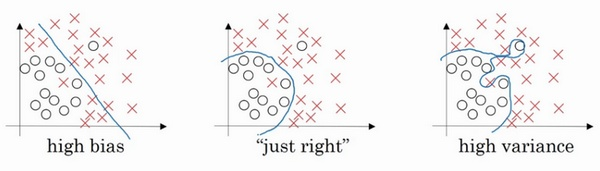1. f(x;D)由训练集 D 学得的模型 f 对 x 的预测输出。
2. y 表示x 的真实值
3. 针对所有训练集，学习算法 f 对测试样本 x 的 期望预测 为:

$\overline{f}(x)=E_D[f(x;D)]$
4. 偏差，偏差度量了学习算法的期望预测与真实结果的偏离程度，即刻画了学习算法本身的拟合能力

$偏差=(\overline{f}(x)-y)^2$
5. 方差，方差度量了同样大小的训练集的变动所导致的学习性能的变化，即刻画了数据扰动所造成的影响

$方差=E_D[(f(x;D)-\overline{f}(x))^2]$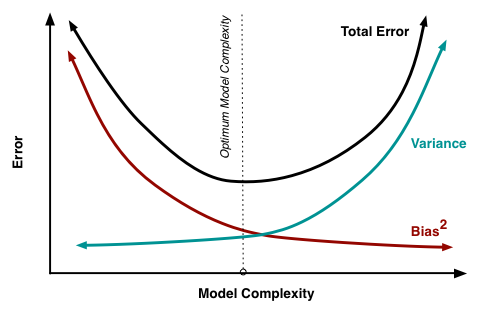1. 给定一个学习任务, 在训练初期, 由于训练不足, 学习器的拟合能力不够强, 偏差比较大, 也是由于拟合能力不强, 数据集的扰动也无法使学习器产生显著变化, 也就是欠拟合的情况;
2. 随着训练程度的加深, 学习器的拟合能力逐渐增强, 训练数据的扰动也能够渐渐被学习器学到;
3. 充分训练后, 学习器的拟合能力已非常强, 训练数据的轻微扰动都会导致学习器发生显著变化, 当训练数据自身的、非全局的特性被学习器学到了, 则将发生过拟合.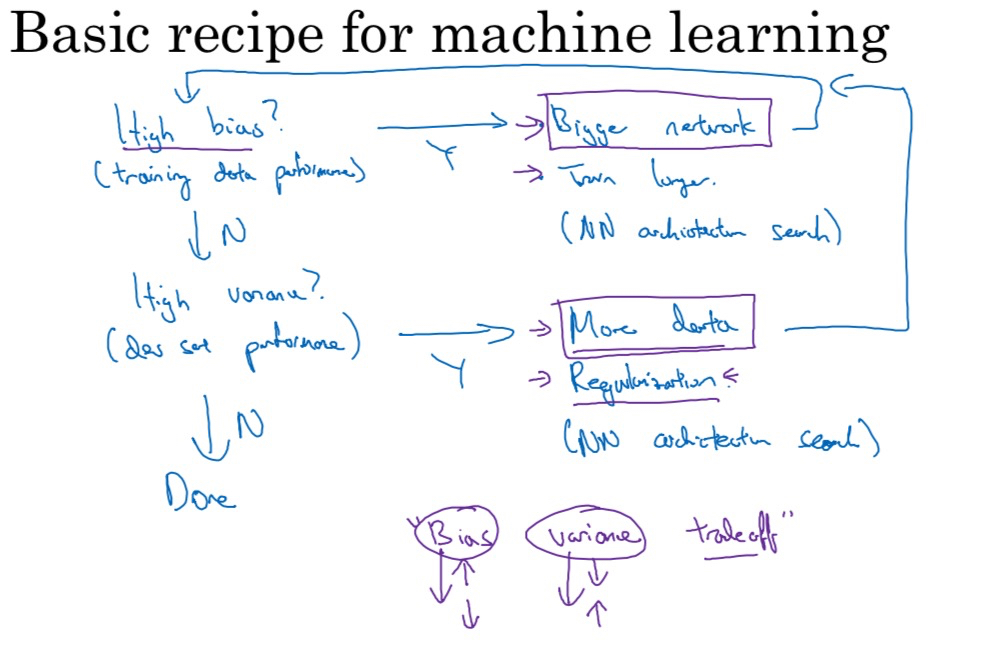1. 初始训练模型完成后，我们首先要知道算法的偏差高不高
2. 如果偏差很高，甚至无法拟合训练集，可以尝试

1. 更大的网络 比如更多的隐层或隐藏单元
2. 花费更多的时间训练算法
3. 更先进的优化算法
4. 新的神经网络结构
5. 准备更多的训练数据对高偏差没啥大用
3. 反复尝试，直到可以拟合训练集，使得偏差降低到可以接受的值
4. 如果方差比较高

1. 更多的训练数据
2. regularization/正则化
3. 新的神经网络结构
5. 不断尝试，直到找到一个低偏差、低方差的框架

## 防止过拟合

1. 简化模型：可以选择较少参数的模型（例如，选择线性模型而不是高阶多项式模型）也可以减少训练数据中的属性数量，或者是约束模型。
2. 收集更多的训练数据。
3. 减少训练数据中的噪声（例如，修复数据错误和消除异常值）。
4. 看一看是不是需要加入 Dropout、正则化项来减轻过拟合的风险。

1. 选择一个带有更多参数、更强大的模型。
2. 给学习算法提供更好的特征集（特征工程）。
3. 减少模型中的约束（例如，减少正则化超参数）。

### 正则化/规则化——给损失函数“加码”

$\begin{Bmatrix} (x^{(i)},y^{(i)}),i=1,...m \end{Bmatrix}$

$$\hat y^i,i =1,...m$$ 损失函数

$J(w,b)=\frac{1}{m}\sum\_{i=1}^mL(\hat y^i , y^i)$

L2正则化是给cost function加上正则项

$J(w,b)=\frac{1}{m}\sum\_{i=1}^mL(\hat y^i , y^i)+\frac{\lambda}{2m}||w||_2^2$

$\frac{d}{d_W}(\frac{\lambda}{2m}W^2)=\frac{\lambda}{m}W$

$$d_w$$ 变得更大，W会变得更小

L1正则化采用的正则化项如下所示

$J(w,b)=\frac{1}{m}\sum\_{i=1}^mL(\hat y^i , y^i)+\frac{\lambda}{2m}||w||_1$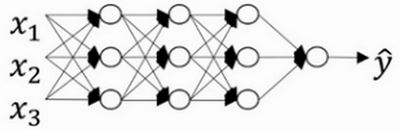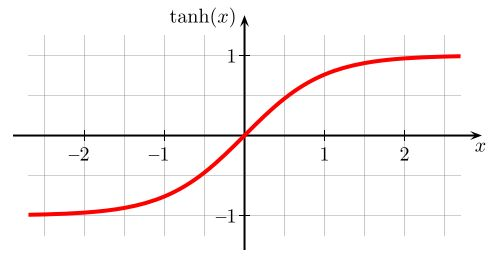## 模型验证

1. 首先是模型性能。模型性能可以理解为模型预测的效果，你可以简单理解为“预测结果准不准”，它的评估方式可以分为两大类：分类模型评估和回归模型评估
1. 分类模型解决的是将一个人或者物体进行分类，例如在风控场景下，区分用户是不是“好人”，或者在图像识别场景下，识别某张图片是不是包含人脸。对于分类模型的性能评估，我们会用到包括召回率、F1、KS、AUC 这些评估指标。
2. 回归模型解决的是预测连续值的问题，如预测房产或者股票的价格，所以我们会用到方差和 MSE 这些指标对回归模型评估。 我们除了要知道可以对模型性能进行评估的指标都有什么，还要知道这些指标值到底在什么范围是合理的。虽然，不同业务的合理值范围不一样，我们要根据自己的业务场景来确定指标预期，但我们至少要知道什么情况是不合理的。比如说，如果算法同学跟我说，AUC 是 0.5，我想都不想就知道，这个模型可能上不了线了，因为 AUC = 0.5 说明这个模型预测的结果没有分辨能力，准确率太差，这和瞎猜得到的结果几乎没啥区别，那这样的指标值就是不合理的。
2. 模型的稳定性，你可以简单理解为模型性能（也就是模型的效果）可以持续多久。我们可以使用 PSI 指标来判断模型的稳定性，如果一个模型的 PSI > 0.2，那它的稳定性就太差了，这就说明算法同学的工作交付不达标。